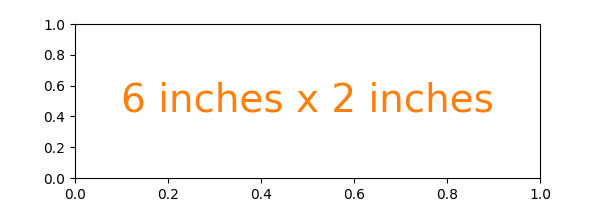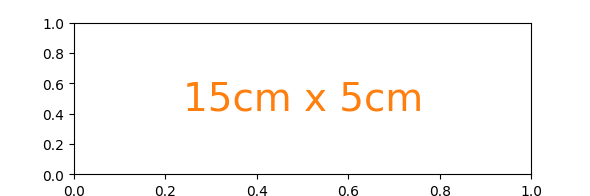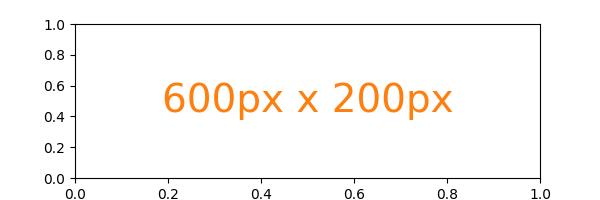# Figure size in different units#

The native figure size unit in Matplotlib is inches, deriving from print industry standards. However, users may need to specify their figures in other units like centimeters or pixels. This example illustrates how to do this efficiently.

import matplotlib.pyplot as plt
text_kwargs = dict(ha='center', va='center', fontsize=28, color='C1')


## Figure size in inches (default)#

plt.subplots(figsize=(6, 2))
plt.text(0.5, 0.5, '6 inches x 2 inches', **text_kwargs)
plt.show()## Figure size in centimeter#

Multiplying centimeter-based numbers with a conversion factor from cm to inches, gives the right numbers. Naming the conversion factor cm makes the conversion almost look like appending a unit to the number, which is nicely readable.

cm = 1/2.54  # centimeters in inches
plt.subplots(figsize=(15*cm, 5*cm))
plt.text(0.5, 0.5, '15cm x 5cm', **text_kwargs)
plt.show()## Figure size in pixel#

Similarly, one can use a conversion from pixels.

Note that you could break this if you use savefig with a different explicit dpi value.

px = 1/plt.rcParams['figure.dpi']  # pixel in inches
plt.subplots(figsize=(600*px, 200*px))
plt.text(0.5, 0.5, '600px x 200px', **text_kwargs)
plt.show()Quick interactive work is usually rendered to the screen, making pixels a good size of unit. But defining the conversion factor may feel a little tedious for quick iterations.

Because of the default rcParams['figure.dpi'] = 100, one can mentally divide the needed pixel value by 100 1:

plt.subplots(figsize=(6, 2))
plt.text(0.5, 0.5, '600px x 200px', **text_kwargs)
plt.show()1

Unfortunately, this does not work well for the matplotlib inline backend in Jupyter because that backend uses a different default of rcParams['figure.dpi'] = 72. Additionally, it saves the figure with bbox_inches='tight', which crops the figure and makes the actual size unpredictable.

References

The use of the following functions, methods, classes and modules is shown in this example:

Keywords: matplotlib code example, codex, python plot, pyplot Gallery generated by Sphinx-Gallery